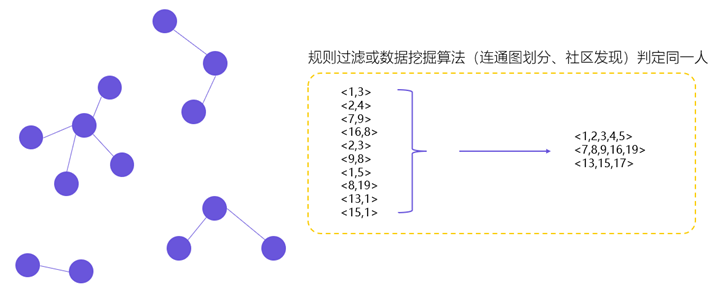# Matplotlib初级入门教程

## Matplotlib简介

### MATLAB

MATLAB是Matrix & Laboratory两个词的组合，意为矩阵工厂（矩阵实验室）。是由美国 Mathworks公司发布的主要面对科学计算、可视化以及交互式程序设计的高科技计算环境。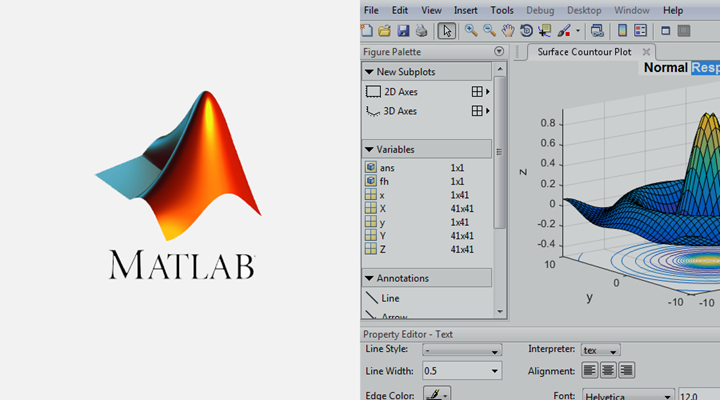### Matplotlib

Matplotlib是Python中使用最广泛的数据可视化库。Matplotlib最早由John Hunter于2002年启动开发，其目的是为了构建一个Matlab式的绘图函数接口。它利用了 Python 下的数值计算模块 Numeric 及 Numarray，克隆了许多 Matlab 中的函数， 用以帮助用户轻松地获得高质量的二维图形。Matplotlib 可以绘制多种形式的图形包括普通的线图，直方图，饼图，散点图以及误差线图等；可以比较方便的定制图形的各种属性比如图线的类型，颜色，粗细，字体的大小等；它能够很好地支持一部分 TeX 排版命令，可以比较美观地显示图形中的数学公式。

Matplotlib 掌握起来也很容易，由于 Matplotlib 使用的大部分函数都与 Matlab 中对应的函数同名，且各种参数的含义，使用方法也一致，这就使得熟悉 Matlab 的用户使用起来感到得心应手。对那些不熟悉的 Matlab 的用户而言，这些函数的意义往往也是一目了然的，因此只要花很少的时间就可以掌握。

• 绘图应该看起来不错 – 发布质量。 对我来说一个重要的要求是文本看起来不错（抗锯齿等）
• 用于包含 TeX 文档的 Postscript 输出
• 可嵌入图形用户界面用于应用程序开发
• 代码应该足够容易，我可以理解它，并扩展它
• 绘图应该很容易

## Matplotlib架构

Matplotlib 架构从逻辑上分为三层，位于三个不同的层级上。上层内容可以与下层内容进行通讯，但下层内容不可以与上层内容通讯。三层从上至下依次是：

• Scripting（脚本层）
• Artist（表现层）
• Backend（后端层）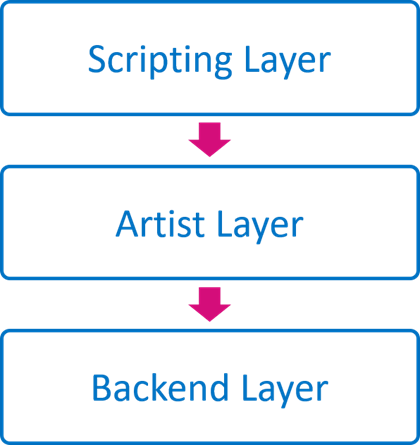### Backend 层

Backend 为最底层，即 matplotlib API 层。Backend在mpl.backend_bases类下，其中包含了各种键盘、鼠标的Event类、用以绘制线段、图形的RenderBase类、负责将Figure对象和其输出分离开的FigureCanvasBase类等（只是一个接口，具体不同输出，比如PDF、PNG的定义在backends类中）。即所有派生/输出的方法都放在mpl.backends下，比如Qt5后端为mpl.backends.qt5agg。输出PDF的后端为mpl.backend_pdf,这里的类负责和不同的后端/平台进行交互。这样的设计保证了代码的解耦和设计的统一。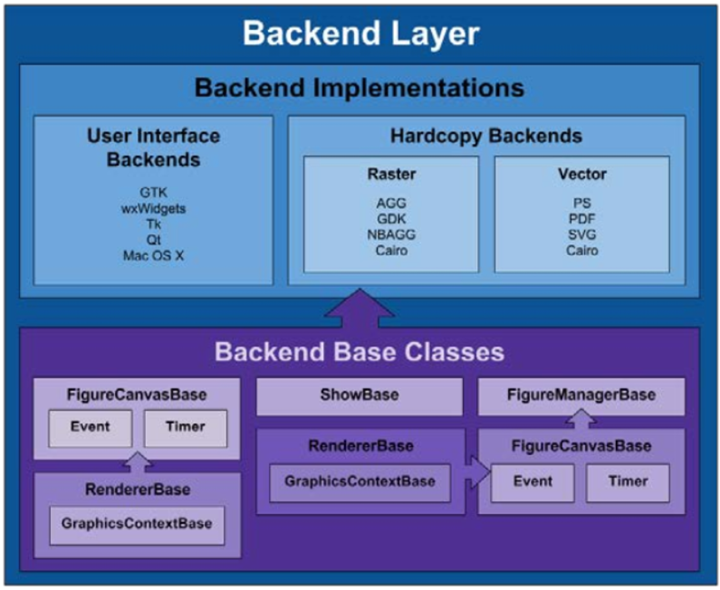• FigureCanves：用来表示图形的绘制区域
• Renderer：用来在 FigureCanves 上绘制内容的对象
• Event：用来处理用户输入（键盘、鼠标输入）的对象

### Artist 层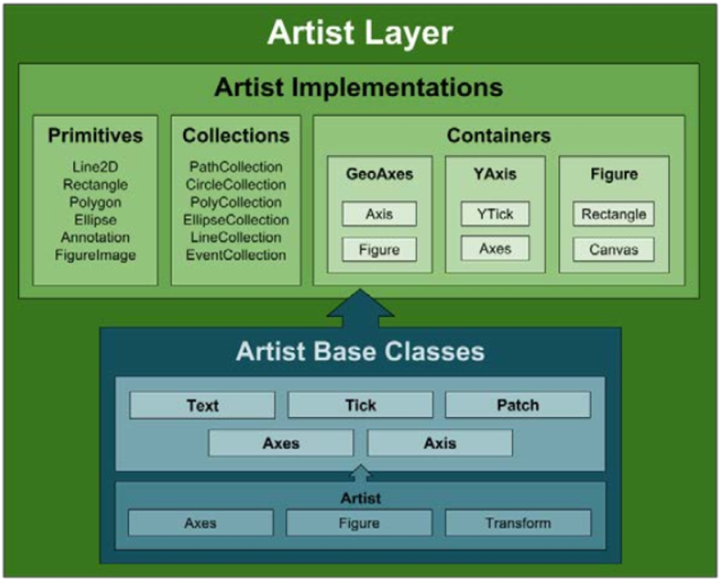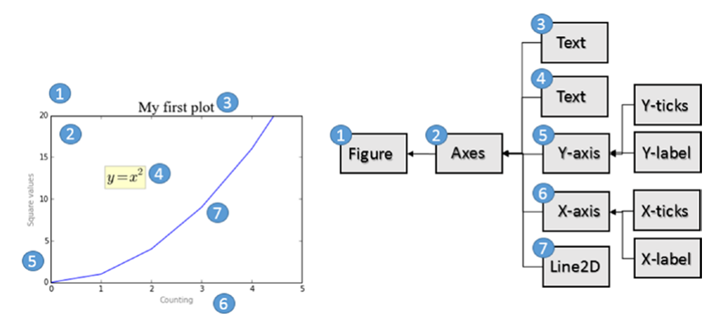Artist 层拥有许多可视化元素，即标题、轴标签、刻度等元素。Artist 分为两类，一类是Primitive Artist，一类是Composite Artist：

• Primitive Artist 是包含基本图形的独立元素，例如一个矩形、一个圆或者是一个文本标签
• Composite Artist 是这些简单元素的组合，比如横坐标、纵坐标、图形等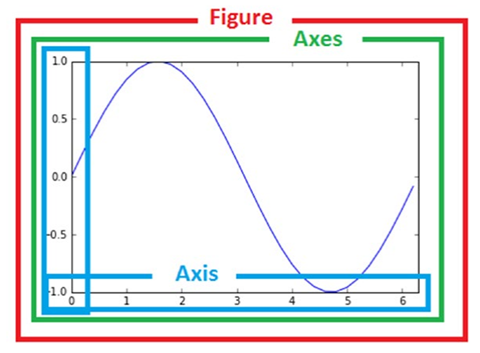Artist 最上层是 Figure 层，表示图形概念； Axes 在 Figure 上面，表示轴的作图的内容（即轴对象），每个维度会有一个 Axes 对象；Axis 用来展示 Axes 上的数值（即刻度与刻度值），刻度的位置用 Locator 对象管理，刻度的标签的格式用 Formatter 对象调整。

### Scripting 层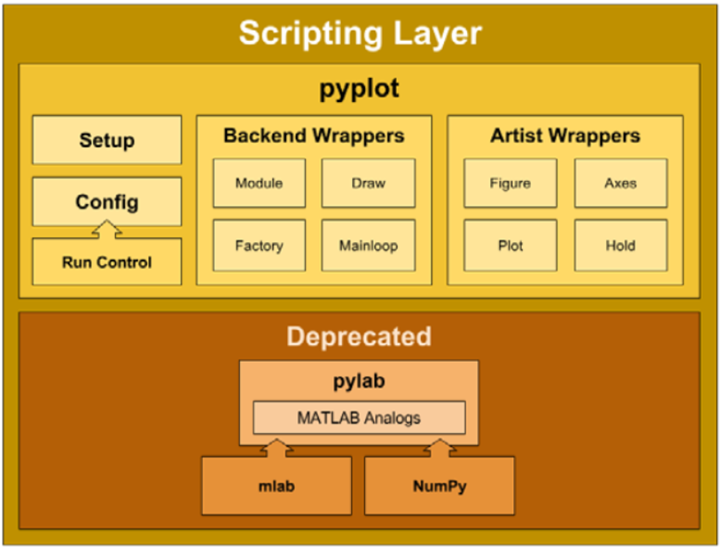### 使用Matplotlib Layers绘制图形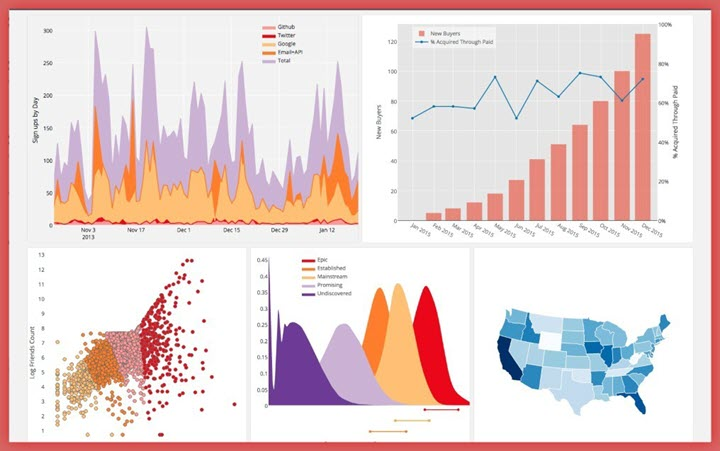# Step One: We import FigureCanvas from Back-end layer.
from matplotlib.backends.backend_agg import FigureCanvasAgg as FigureCanvas

# Step two: We import Figure Artist
from matplotlib.figure import Figure

# Step 3: We create a Figure object using the constructor
fig = Figure()

import numpy as np
np.random.seed(6)
x = np.random.randn(20000)

# Next we create an Axes Artist object.

# Next we call the Axes method hist() function.
ax.hist(x, 100)

# Finally we assign a title to the plot.
ax.set_title('Artist Layer Histogram')

# And we save it, in this case as a PNG file.
fig.savefig('Matplotlib_histogram.png')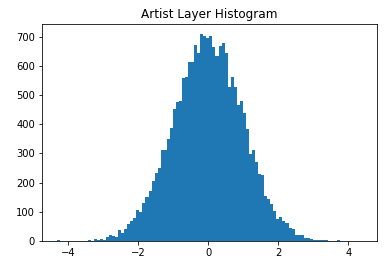• ax.hist(x,100)：为每个柱状图条创建一个矩形原始Artistlayer对象序列，并将它们添加到ExesContainer中。100个意味着产生100个柱状图条。

import matplotlib as mpl
import matplotlib.pyplot as plt
import numpy as np

np.random.seed(6)
X = np.random.randn(20000)

plt.hist(X, 100)
plt.title('Scripting Layer Histogram')
plt.savefig('matplotlib_histogram.jpg')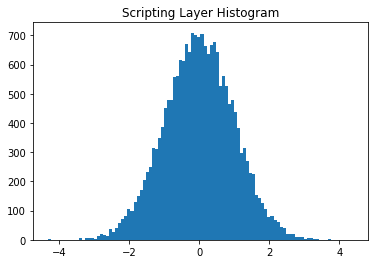Artist 还是 Scripting Layer?

## Matplotlib图像结构

• Canvas（画板）位于最底层，用户一般接触不到
• Figure（画布）建立在Canvas之上
• Axes（绘图区）建立在Figure之上
• 坐标轴（axis）、图例（legend）等辅助显示层以及图像层都是建立在Axes之上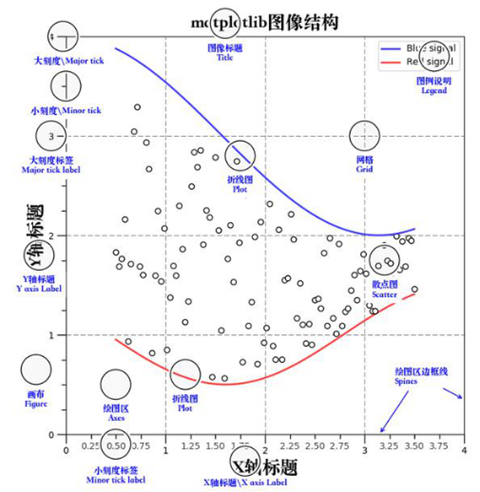### 容器层

• Canvas（画布）底层的系统层，在绘图的过程中充当画板的角色，即放置Figure（绘图区）的工具
• Figure是Canvas上方的第一层，也是需要用户来操作的应用层的第一层，在绘图的过程中充当画布的角色。
• Axes是应用层的第二层，在绘图的过程中相当于画布上的绘图区的角色。
• Figure:指整个图形(可以通过figure()设置画布的大小和分辨率等)
• Axes(坐标系):数据的绘图区域
• Axis(坐标轴)：坐标系中的一条轴，包含大小限制、刻度和刻度标签

• 一个figure(画布)可以包含多个axes(坐标系/绘图区)，但是一个axes只能属于一个figure。
• 一个axes(坐标系/绘图区)可以包含多个axis(坐标轴)，包含两个即为2d坐标系，3个即为3d坐标系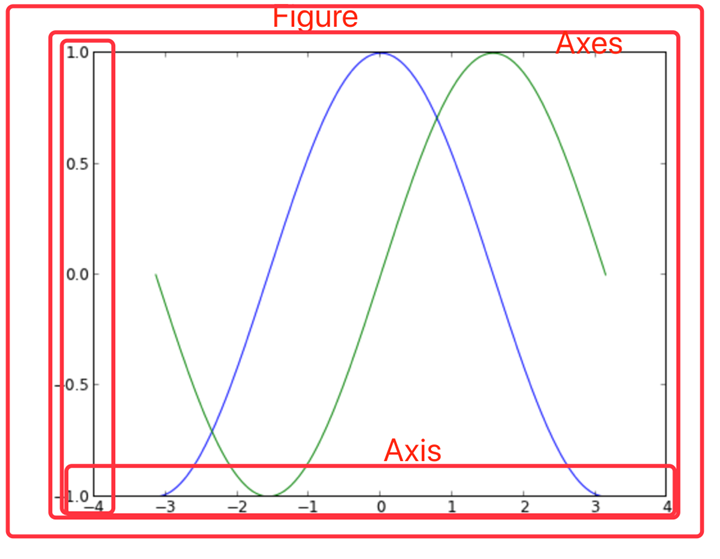### 辅助显示层

• Axes外观(facecolor)
• 边框线(spines)
• 坐标轴(axis)
• 坐标轴名称(axis label)
• 坐标轴刻度(tick)
• 坐标轴刻度标签(tick label)
• 网格线(grid)
• 图例(legend)
• 标题(title)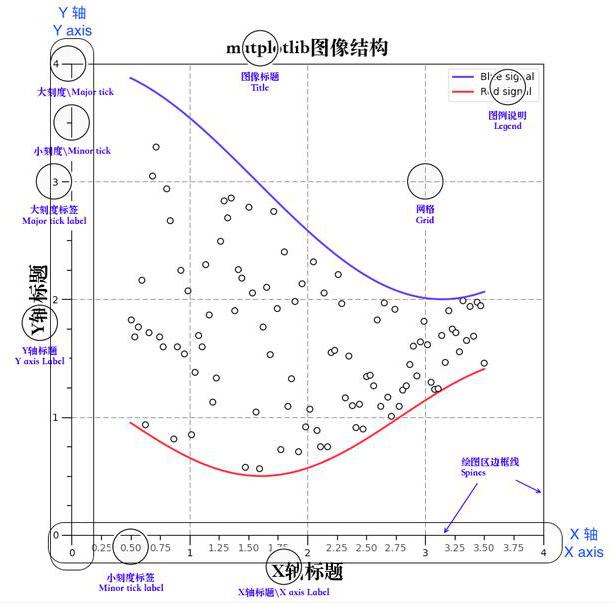### 图像层

• plot（折线图）
• scatter（散点图）
• bar（柱状图）
• histogram（直方图）
• pie（饼图）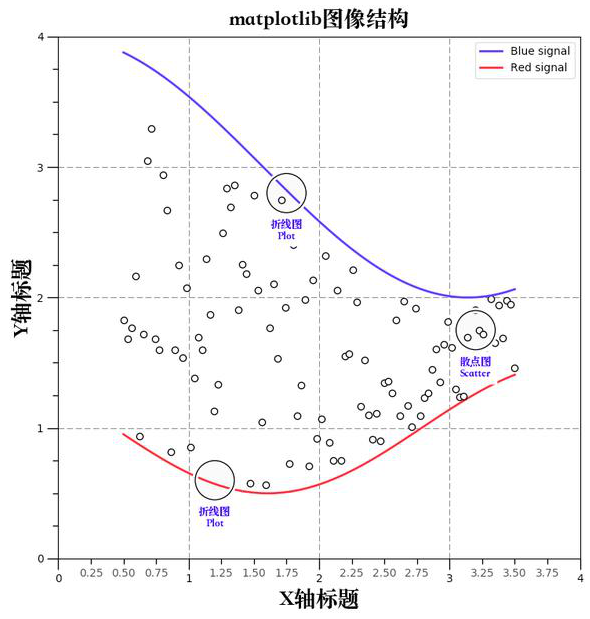## 使用PYPLOT进行绘图

### plt.plot()绘图

plt.plot(np.random.randn(50).cumsum(), 'r--.')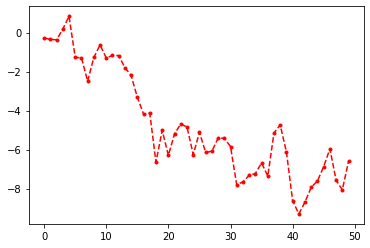pyplot.plot 可以接受绘制对象和一些类似于命令行的参数，可选颜色、点线状态（水平线、垂直线、星标）等各种形状。plot的参数参见Line 2D，可以这样指定：

plot(x, y, color='green', linestyle='dashed', marker='o',markerfacecolor='blue', markersize=12)

pyplot可以直接按照面向过程方式处理，调用plt.axis会对当前Axes中的axis属性进行设置。

import matplotlib.pyplot as plt

plt.plot([1,2,3,4], [2,21,23,11], "r^", [12,21,43,21], "b", [0,2,12,40,18], "y--")#x轴、y轴和其颜色、类型、第二个y轴和其颜色、类型、第三个y轴和其颜色、类型
plt.title("My First plt Figure")
plt.axis([-1,5,-10,50]) #sets the min and max of the x and y axes, with v = [xmin, xmax, ymin, ymax]
plt.show()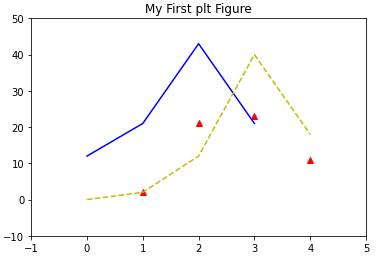• subplot(m,n,a) 在一个Figure和Axes上绘制多个图形，你可以在任何时候返回这个子图进行修改。
• title() 设置标题
• grid() 设置网格
• xlabel() 设置x轴标签
• xticks() 设置x轴刻度
• legend() 控制图例显示
• text() 在指定位置放置文本
from matplotlib.ticker import NullFormatter
import numpy as np

# 设置x轴和y轴的数据
t = np.arange(0, 5, 0.1)
y1 = np.sin(2 * np.pi * t)
y2 = np.sin(2 * np.pi * t)

# 设置整体图形外观
plt.subplots_adjust(top=2, bottom=0.08, left=0.10, right=0.95, hspace=0.25, wspace=0.35)

# plt.title("My First plot") not work here
# 设置第一个子图形的标题、绘图、标签、网格
plt.subplot(411)  # 用来水平切分4个，垂直切分1个，调用第1个图形
plt.title("My First plot", fontsize=14)  # only work here
plt.plot(t, y1, "b-.")  # 在这里还可以设置绘制的点的样式、颜色等
plt.ylabel("Value-M1")
plt.grid(True)

# 同上，调用第二个图形，设置文本和图例
plt.subplot(412)
plt.plot(t, y2, "r--")
plt.ylabel("Value-M2")
plt.text(1, 0.5, "text1")
plt.text(3, 0.3, "text2")
plt.legend(["Legend"], loc=1)  # 图例位置和文本内容

# 同上，调用第三个图形，gca返回当前instance，设置刻度
plt.subplot(413)
plt.plot(t, y1, "p-.")
plt.gca().xaxis.set_minor_formatter(NullFormatter())  # gca获取当前axes，set..设置刻度

plt.subplot(411)
# 你可以在任意时候，回到之前绘制的子图中继续在其上绘制其他图案。比如：
# plt.plot(t,y1,"p-.") #这条命令会绘制在图1中，并且和之前图1存在的内容并存
plt.show()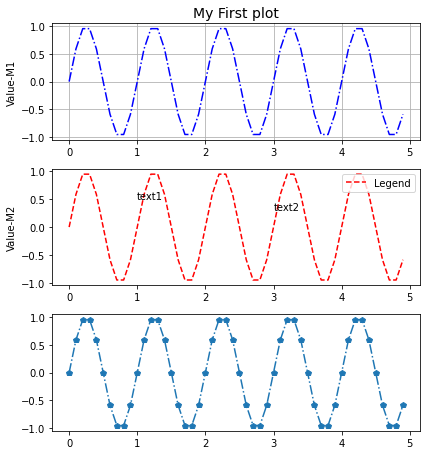### Figure和Axes：图形、子图形和其轴

Figure和Axes对象

class matplotlib.figure.Figure
(figsize=None, dpi=None, facecolor=None, edgecolor=None, linewidth=0.0, frameon=None, subplotpars=None, tight_layout=None)


Figure还有很多方法，常有的有：

• clear() 清除图像
• draw(renderer) 底层接口
• gca() 当前Axes
• get_children() 获取子元素
• hold() 丑陋的MATLAB兼容性
• savefig() 保存图片
• set_canvas() 底层接口
• show() 显示图片
• text() 放置文字
class matplotlib.axes.Axes
(fig, rect, facecolor=None, frameon=True, sharex=None, sharey=None, label='', xscale=None, yscale=None, axisbg=None, **kwargs)


Axes有很多方法，单独方法大约有100种，因此在这里不再举例，可查阅手册。

• gca() 返回当前状态下的Axes对象，Fig.gca()的Alias
• gca().get_children() 方便查看当前Axes下的元素
• gcf() 返回当前状态下的Figure对象,一般用以遍历多个图形的Axes(plt.gcf().get_axes())。另一种方法是使用Axes矩阵的索引抽取子Plot的Axes。

• axes = plt.subplot(mna) #MATLAB方式，一般仅用作交互，而不捕获输出
• axes = mpl.axes.Axes(fig,*kwargs #OOP方式，较少使用
• fig, axes_martix = plt.subplots(nc,nr) #快速方式，适合快速创建Figure对象和Axes对象，一般用于创作指定子图形个数的图。

from mpl_toolkits.mplot3d import Axes3D

fig = plt.figure() #调用一个新的画布
fig2 = plt.gcf() # fig2 == fig1 => True

ax_sub = fig.add_subplot(2, 2, 1) #添加子画布，2×2大小，第一个
ax_sub2 = fig.add_subplot(2, 2, 2) #添加子画布，2×2大小，第二个
ax_sub3 = fig.add_subplot(2, 2, 3) #添加子画布，2×2大小，第三个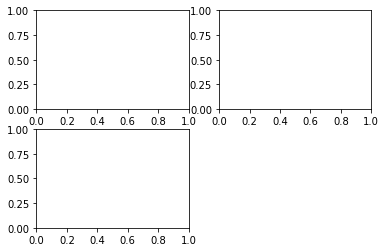%matplotlib inline
x = np.arange(0.1, 4, 0.5)
y = np.exp(-x)
#快速创建图形 Fig 和 Axes Martix
fig, ax = plt.subplots(3, 3, sharex=True, sharey=True)
#或者使用 fig, ((ax1,ax2,ax3),(ax4,ax5,ax6),(ax7,ax8,ax9)) = plt.subplots(3,3)
print(ax)

# plot the linear_data on the 5th,8th subplot axes
ax.plot(x,y, '-')
ax.plot(x,y**2, '-')

# 对于一个包含多个轴的fig，可以使用 gcf().get_axis，或者直接对矩阵进行索引和操纵
for ax in plt.gcf().get_axes():
for label in ax.get_xticklabels() + ax.get_yticklabels():
label.set_visible(True)

# necessary on some systems to update the plot
plt.gcf().canvas.draw()

[[<matplotlib.axes._subplots.AxesSubplot object at 0x7f57f8c3b310>
<matplotlib.axes._subplots.AxesSubplot object at 0x7f57f8bec110>
<matplotlib.axes._subplots.AxesSubplot object at 0x7f57f86c11d0>]
[<matplotlib.axes._subplots.AxesSubplot object at 0x7f57f8b16290>
<matplotlib.axes._subplots.AxesSubplot object at 0x7f57f84e7350>
<matplotlib.axes._subplots.AxesSubplot object at 0x7f57f86ed410>]
[<matplotlib.axes._subplots.AxesSubplot object at 0x7f57f8ca8a10>
<matplotlib.axes._subplots.AxesSubplot object at 0x7f57f857ecd0>
<matplotlib.axes._subplots.AxesSubplot object at 0x7f57f855ce50>]]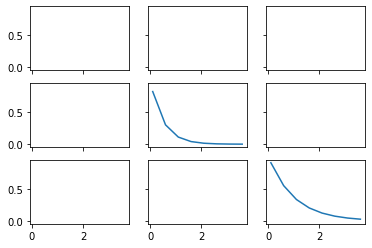### 绘图常用方法

Firure常用方法

• savefig(fname,dpi,bbox_inches,format)保存图像

Axes常用方法

• hist 柱状图/pie 饼状图/box 箱型图等等
• plot 如果不指定图的类型，比如饼状图或者柱状图，直接传入plot，参数填为data即可。
• annotate 添加箭头
• text 添加文本说明
• get(set)_x(y)lim 获得/设置不同轴的范围
• g/s_(x/y)ticks 设置刻度位置 #plt.xticks((0,1,2),(“a”,”b”,”c”)) 可以快速指定位置和标签值。
• g/s_(x/y)ticklabels 设置刻度标签
• g/s_(x/y)label 设置轴名称
• title 题头设置
• legend 图例设置

fig, ax = plt.subplots(2, 3, sharex=True, sharey=True)
#也可以使用plt顶级函数
#histogram 柱状图
ax[0,0].hist(np.random.randint(1,500,size=100),bins=50,color="k",alpha=0.5)
ax[0,1].hist(np.random.randint(1,500,size=100),bins=50,color="k",alpha=0.5)
ax[0,2].hist(np.random.randint(1,500,size=100),bins=50,color="k",alpha=0.5)
ax[1,0].hist(np.random.randint(1,500,size=100),bins=50,color="k",alpha=0.5)
ax[1,1].hist(np.random.randint(1,500,size=100),bins=50,color="k",alpha=0.5)
ax[1,2].hist(np.random.randint(1,500,size=100),bins=50,color="k",alpha=0.5)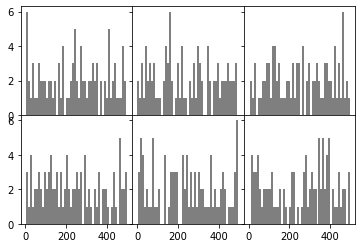fig.savefig("hello.png",format="png", dpi=100,bbox_inches="tight")
#两个较为重要的参数是bbox_inches控制边距，第二个是dpi控制精度

fig,ax = plt.subplots()
ax.plot(np.random.randn(500).cumsum(),label="a")
#此处设置plot的label值，即可以调用legend时自动生成图例。
ax.plot(np.random.randn(500).cumsum(),label="b")
ax.set_xticks([0,150,300,450,600])
ax.set_xticklabels(["one","two","three","four","five","six"]) #自动跳过了six
#也可以直接写ax.xticks([真实数值],[对应标签名称])
ax.set_title("table",loc="center")
ax.set_xlabel("numbers")
ax.set_ylabel("values")
ax.legend(loc="best") #legend图例，在plot时传入label进行创建
ax.text(0,10,"Hello World")
# matplotlib.pyplot.text(x, y, s, fontdict=None, withdash=False, **kwargs)
ax.annotate("", xy=(0, 0), xycoords='data', xytext=(400,10), textcoords='data', arrowprops=dict(arrowstyle="->", connectionstyle="arc3"),)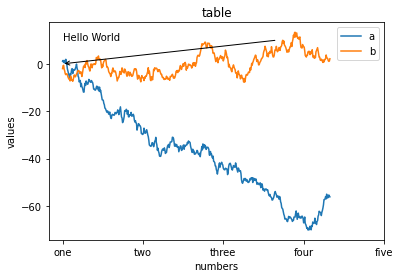SPINES坐标系绘制

%matplotlib inline
fig ,ax = plt.subplots()
x = np.arange(-2*np.pi,2*np.pi,0.01)
y = np.sin(3*x)/x
y2 = np.sin(2*x)/x
y3 = np.sin(x)/x

ax.plot(x,y,"r")
ax.plot(x,y2,"b")
ax.plot(x,y3,"g")

print(plt.gca(),ax)
# AxesSubplot(0.125,0.125;0.775x0.755) AxesSubplot(0.125,0.125;0.775x0.755)

ax.spines["right"].set_visible(False)
#class matplotlib.spines.Spine(axes, spine_type, path, **kwargs)
ax.spines["top"].set_visible(False)
ax.spines["bottom"].set_position(("data",0))
ax.spines["left"].set_position(("data",0))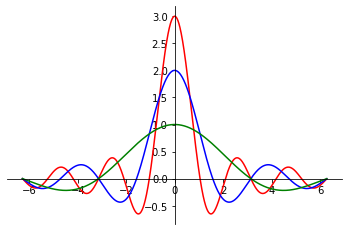PATCH图形的绘制

import matplotlib.path as mpath
import matplotlib.patches as mpatches
fig = plt.figure(figsize=(12,6))
grid = np.mgrid[0.2:0.8:3j, 0.2:0.8:3j].reshape(2, -1).T
patches = []
circle = mpatches.Circle(grid, 0.1, ec="none")
patches.append(circle)
rect = mpatches.Rectangle(grid - [0.025, 0.05], 0.05, 0.1, ec="none")
patches.append(rect)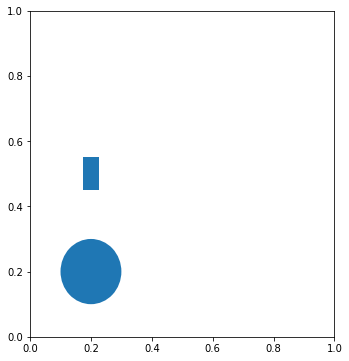AXES3D图表绘制

from mpl_toolkits.mplot3d import Axes3D

x = np.arange(8)#生成数据
y = np.random.randint(0,10,8)
y2 = y + np.random.randint(0,3,8)
y3 = y2 + np.random.randint(0,3,8)
y4 = y3 + np.random.randint(0,3,8)
y5 = y4 + np.random.randint(0,3,8)

fig = plt.figure() ; ax = Axes3D(fig) #普通方法调用fig和ax
# For those using older versions of matplotlib,
#change ax = fig.add_subplot(111, projection='3d') to ax = Axes3D(fig).

ax.bar(x,y,0,zdir="y")#数据和作为第三轴的轴：y
ax.bar(x,y2,10,zdir="y")
ax.bar(x,y3,20,zdir="y")
ax.bar(x,y4,30,zdir="y")
ax.bar(x,y5,40,zdir="y")
ax.set_xlabel("X label")#标签
ax.set_ylabel("Y label")
ax.set_zlabel("Z label")
ax.view_init(elev=50)#视角
ax.grid(False)
#3D设置在class mpl_toolkits.mplot3d.axes3d.Axes3D(fig, rect=None, *args, **kwargs)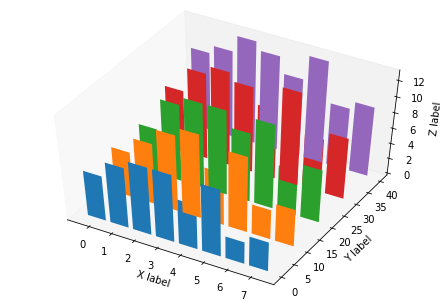fig = plt.figure()
ax_1 = fig.add_axes([0.1,0.1,1,1]) #此处定义的是图表的 rect [left, bottom, width, height]
ax_1.plot(np.random.randint(0,10,50),"ro")
ax_2.plot(np.random.randint(0,10,3),"g--")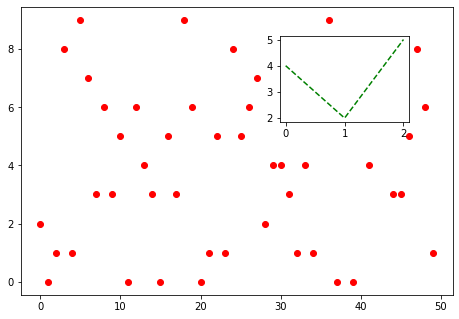fig = plt.figure()
gs = plt.GridSpec(3,3) #新建一个网格
s2.plot(np.random.randint(0,10,10),"o")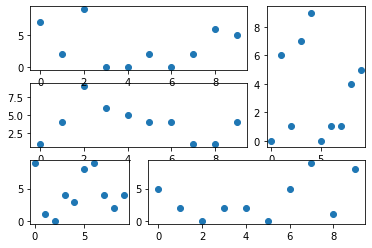from matplotlib.gridspec import GridSpec
import numpy as np

fig = plt.figure()
gs = GridSpec(3,3)

data_x = np.random.random(size=10000)
data_y = np.random.normal(size=10000)

main.scatter(data_x,data_y)
main.set_xlim(0,1)
hist.hist(data_y,orientation='horizontal',bins=1000) #水平显示
hist.invert_xaxis() #反转X轴以方便比较数据
hist_x.hist(data_x,bins=1000)
hist_x.set_xlim(0,1)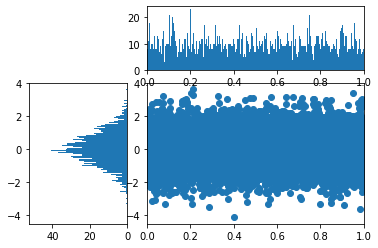GCA高级用法

plt.gca().fill_between(x,y1,y2,alpha,color)

xaxis = plt.gca().get_xaxis() #从AXES实例中获取XAXIS实例

for item in xaxis.get_ticklabels(): #从XAXIS实例中获取TICKLABELS
item.set_alpha(1) #TICKLABELS由TEXT实例构成，TEXT实例有很多方法
item.set_rotation(45)
item.set_backgroundcolor('r')
item.set_color('w')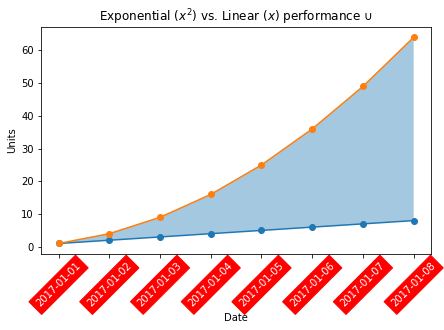gca()本质上是一个Axes对象，查阅文档可以看出，这个对象具有很多可用的方法，比如本例中取出x子轴，并且对此轴的刻度标签进行遍历（Text对象），设置旋转、透明度和颜色、背景，对于较长的时间日期，这样可以使得坐标轴文字不挤在一起。

## Matplotlib样式

import matplotlib.pyplot as plt
print(plt.style.available)


import matplotlib.pyplot as plt
plt.style.use('ggplot')
# 使用自定义样式：
plt.style.use('./images/presentation.mplstyle')
plt.style.use('https://github.com/dhaitz/matplotlib-stylesheets/raw/master/pitayasmoothie-light.mplstyle')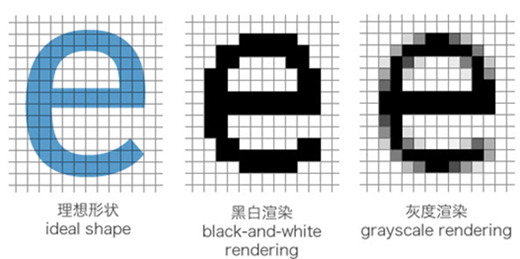##### 网站与APP开发中的字体设置##### 如何理解Python装饰器